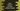# Java program to check if a number is Nelson number or not## Java program to check if a number is Nelson number or not:

In this post, we will learn how to check if a number is Nelson number or not. This program will take one number as input from the user, check if it is a Nelson number and print one message.

### What is a Nelson number:

In cricket, 111 is known as Nelson number and a score of 111 is considered as unlucky. It is named after Admiral Nelson, who allegedly only had one arm, one leg and one eye near the end of his life.

Multiple of 111 is also a Nelson number. These are known as double Nelson for 222, triple Nelson for 333, quadruple Nelson for 444 etc.

So, if we want to check if a number is Nelson or not programmatically, we have to check if the remainder is 0 if we divide the number by 111. We can use modulo operator for this.

### Modulo operator:

% or modulo operator gives the remainder value. For example, first % second will give the remainder if we divide first by second.

i.e. if number is a Nelson number, number % 111 will be 0 always. Let’s use it in a Java program:

### Java program to check if a user-given number is Nelson or not:

Let’s take a look at the below program:

``````import java.util.Scanner;

public class Main {

public static void main(String[] args) {
int num;
Scanner sc = new Scanner(System.in);

System.out.println("Enter a number: ");
num = sc.nextInt();

if (num % 111 == 0) {
System.out.println(num + " is a Nelson number.");
} else {
System.out.println(num + " is not a Nelson number.");
}

}
}``````

Here,

• num is an integer value to hold the user input value.
• sc is a Scanner object. This object is used to read the user input.
• It asks the user to enter a number, reads it and stores that in num.
• The if block is checking if the entered number is divisible by 111 or not.
• If yes, it is a Nelson number. Else it is not a Nelson number. Based on the result, it prints a message.

If you run this program, it will print output as like below:

``````Enter a number:
223
223 is not a Nelson number.

Enter a number:
333
333 is a Nelson number.``````

### Java program to check if a user-given number is Nelson or not by using a different method:

We can also use a separate method to do the checking, i.e. the method will check if a number is Nelson or not. It will take one number as the parameter, check if that number is Nelson or not and return one boolean value.

Below is the complete program:

``````import java.util.Scanner;

public class Main {

public static boolean isNelson(int num) {
return num % 111 == 0;
}

public static void main(String[] args) {
int num;
Scanner sc = new Scanner(System.in);

System.out.println("Enter a number: ");
num = sc.nextInt();

if (isNelson(num)) {
System.out.println(num + " is a Nelson number.");
} else {
System.out.println(num + " is not a Nelson number.");
}

}
}``````

In this example,

• We have created another method called isNelson.
• isNelson method takes an integer number as the input and returns one boolean value.
• It returns true if the number passed in the parameter is a nelson number. Else, it returns false.
• We are calling this method to check if the number num is a nelson number or not. Based on its return value, it is printing a message.

If you run this program, it will print similar output as like below:

``````Enter a number:
333
333 is a Nelson number.``````

Using a separate method is useful always because we can call this method from any other class as well. By using a separate method, we can call this method from a different class and it will return the same output.